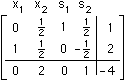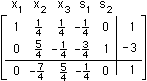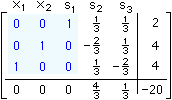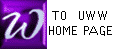IDENTITY MATRICES
IDENTITY SUB-MATRIX (ISM)

IDENTITY MATRICES perform for matrix arithmetic what the number "1" performs for the arithmetic of numbers. Below are the first four identity matrices.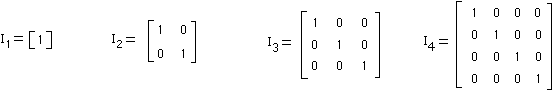An IDENTITY SUB-MATRIX (ISM) is an identity matrix contained in a larger matrix. An ISM within a Simplex Tableau may appear with it's columns permuted (re-arranged) into an order different from the order shown above. For example, below are 3 matrices from a discussion of SIMPLEX METHOD

In  the ISM is in columns x1 and s1;
In  the ISM is in columns x1 and s2;
In  the ISM in in columns x1, x2, s1.